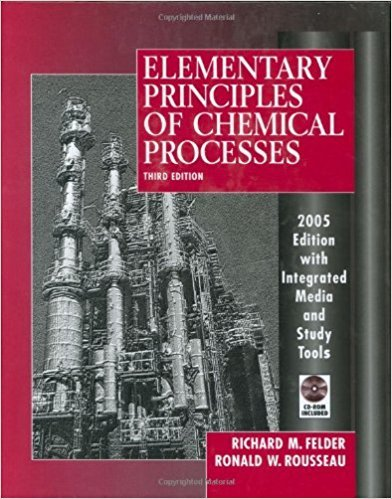×
Get Full Access to Elementary Principles Of Chemical Processes - 3 Edition - Chapter 6 - Problem 6.48
Get Full Access to Elementary Principles Of Chemical Processes - 3 Edition - Chapter 6 - Problem 6.48

×

# The pressure in a vessel containing methane and water atISBN: 9780471687573 143

## Solution for problem 6.48 Chapter 6

Elementary Principles of Chemical Processes | 3rd Edition

• Textbook Solutions
• 2901 Step-by-step solutions solved by professors and subject experts
• Get 24/7 help from StudySoup virtual teaching assistantsElementary Principles of Chemical Processes | 3rd Edition

4 5 1 276 Reviews
27
3
Problem 6.48

The pressure in a vessel containing methane and water at 70C is 10 atm. At the given temperature. the Henry's law constant for methane is 6.66 X 104 atm/mole fraction. Estimate the mole fraction of methane in the liquid

Step-by-Step Solution:
Step 1 of 3

Step 2 of 3

Step 3 of 3

##### ISBN: 9780471687573

This textbook survival guide was created for the textbook: Elementary Principles of Chemical Processes, edition: 3. This full solution covers the following key subjects: methane, atm, Mole, fraction, Law. This expansive textbook survival guide covers 13 chapters, and 710 solutions. Elementary Principles of Chemical Processes was written by and is associated to the ISBN: 9780471687573. The answer to “The pressure in a vessel containing methane and water at 70C is 10 atm. At the given temperature. the Henry's law constant for methane is 6.66 X 104 atm/mole fraction. Estimate the mole fraction of methane in the liquid” is broken down into a number of easy to follow steps, and 39 words. The full step-by-step solution to problem: 6.48 from chapter: 6 was answered by , our top Chemistry solution expert on 11/15/17, 02:42PM. Since the solution to 6.48 from 6 chapter was answered, more than 463 students have viewed the full step-by-step answer.

Unlock Textbook Solution